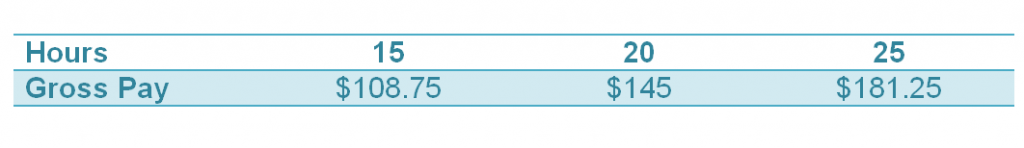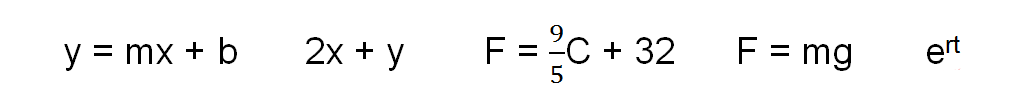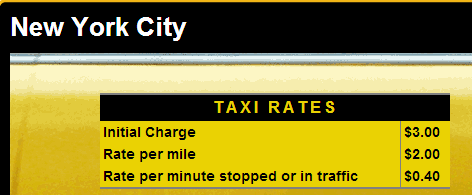# Equations and Expressions

//Equations and Expressions
Equations and Expressions 2017-11-13T22:01:53+00:00

Stacie Bender 8/17/14

Algebra, like any subject, can seem like a foreign language if you don’t pay attention to the terminology. In Algebra or Pre-Algebra, you move from concrete math to something that’s more abstract. Algebra introduces the concept of a variable or a quantity that is unknown.

### Example 1

Over the course of three weeks at her job, Alyssa earned the following amounts:p = 7.25h

Her pay depends on how many hours she works. Her hours vary based on how busy the company she works for is, staffing levels, if she’s available for a shift and other factors. How would we determine how much she makes per hour? We can do that out by dividing her pay by the number of hours she works. She is payed \$7.25/hour. Now we can write an equation about her wages.

7.25 is a constant. It is a number that does not change in our equation..

h is a variable that stands for hours in this case. There is an implied times sign between the constant and the variable. In Algebra we drop the times sign because we don’t want to confuse it with the variable x.

Whenever you see an equals sign you are working with an equation. Think of an equation as a sentence. It is a complete thought. In fact, it’s often possible to substitute the word is for the equal sign.

An expression is like a phrase; it is an incomplete thought.

Can you identify the expressions in this example?### Example 2

Defy Gravity, an indoor trampoline park, charges by the half hour for open jumping and dodge ball based on the expression below. Use the expression to fill out the table.

10t + 3.5

 30 minutes (.5 hours) 1 hour 90 minutes (1.5 hours) 2 hours ?

### Example 3

How could we write an expression that explains how taxi rates work in New York City?First, what’s our constant – the charge that doesn’t change?

The minute we close the door on the cab, we have agreed to pay \$3.

What are our variables? What changes?

The mileage changes and the time we spend in traffic varies. We have to take care here to choose our variables carefully. Even though we’d like to use m for miles and m for minutes, we need two different variables. Unless you are told what to use for your variables, there is no definitive answer. Your expression may be 3 + 2m + .4t where m stands for miles and t is the time stopped or in traffic. One of your classmates may choose 3 + 2x + .4y. If your teacher asks you to declare your variables, he/she wants to make sure everyone knows what each variable stands for so there is no confusion.

Remember that variables are unknowns. Expressions are incomplete thoughts. Equations are complete sentences.

Much of Algebra is centered around solving equations. An equation is solved when the value found for the variable makes the equation true.

### Example 4

What value for x makes the following equation true?

3x – 5 = 28

At the moment, the only tools you have in your bag of tricks are

1. make a table trying each value for x until you find the correct answer or
2. make educated guesses and check to see if you are right. Mentally ask yourself “3 times what number subtracting 5 is 28”?

If we substitute 10 in for x, is the equation true?

3(10) – 5 = 28

Does 30 – 5 = 28?

no, 25 doesn’t equal 28 so x is not 10. We are close but we need a larger result so we can try to substitute 11 for x.

3(11) – 5 = 28

33 – 5 = 28 is true so x = 11.

It’s not a very efficient way to solve equations, but it is a start. Stay tuned. We’ll add to our bag of tricks soon.

Text:

Holt, Rinehart and Winston Algebra 1 p. 11 – 17